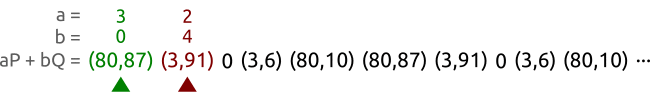## 0x00 前言

### 参考的网站

• https://andrea.corbellini.name/2015/06/08/elliptic-curve-cryptography-breaking-security-and-a-comparison-with-rsa/
• https://github.com/p4-team

### 相关代码

• 本文的完整代码
• https://github.com/ninthDevilHAUNSTER/ecc_learning

## 0x01 BSGS 小步大步法

### 1.1 算法简介

BSGS方法的步骤就是中间相遇。算是一种比较机智的暴力搜索法。该算法的工作步骤如下。

• 计算
• 对于 ，计算并打表
• 对于
• 计算
• 寻找与 上式子结果相同的
• 如果找到，那么• 对于 这样就去遍历了所有 的情况
• 对于 这样就去遍历了所有 的情况
• ...
• 对于 这样就去遍历了所有 的情况

### 1.2 算法实践

def BSGS(E, p, q):
# B
step = 0
hash_table = {}
m = math.floor(math.sqrt(E.GF))
for i in range(m):
tmp = E.get_scalar_multiplication(i, p)
hash_table[tmp] = i
hash_list = hash_table.keys()
# G
for a in range(m):
amP = E.get_scalar_multiplication(-a * m, p)
Q_amP = E.get_three_pionts(amP, q)
if Q_amP in hash_list:
return hash_table[Q_amP] + a * m, step
else:
step += 1
return 0, 0


    E = EllipticCurve(a=1, b=-1, p=10177, GF=10331)
p = (0x1, 0x1)
q = (0x1a28, 0x8fb)
k = 325
k0, step = BSGS(E, p, q)


## 0x02 Pollard rho 算法

### 2.1 计算循环序列### 2.2 算法实践

    E = EllipticCurve(a=1, b=-1, p=10177, GF=10331)
p = (0x1, 0x1)
q = (0x1a28, 0x8fb)
k = 325
print(log(p, q, E))
(325, 221)


## 0x04 SageMath 工具

sage: E =EllipticCurve(GF(10177),[1,-1])
sage: P = E([1,1])
sage: Q = E([0x1a28,0x8fb])
sage: P.discrete_log(Q)
325
# 这算的速度，绝对是秒级别的


## 0x05 ECC VS RSA

RSA key size (bits) ECC key size (bits)
1024 160
2048 224
3072 256
7680 384
15360 521

## 0x06 CTF题目实战

### XUSTCTF 2016

已知椭圆曲线加密Ep(a,b)参数为

p = 15424654874903
a = 16546484
b = 4548674875
G(6478678675,5636379357093)

k = 546768



from curve_base_class import EllipticCurve
E = EllipticCurve(p=15424654874903, a=16546484, b=4548674875)
K = E.get_scalar_multiplication(546768,(6478678675,5636379357093))
print("XUSTCTF{%s}"%(K+K))


### HXP 2018 Curve12833227

WP:https://github.com/p4-team/ctf/blob/master/2018-12-08-hxp/crypto_curve12833227/vuln.py

from random import randrange
from Crypto.Cipher import AES

p = 2**128 - 33227

i = lambda x: pow(x, p-2, p)

x = randrange(p)
aes = AES.new(x.to_bytes(16, 'big'), AES.MODE_CBC, bytes(16))
cipher = aes.encrypt(flag.ljust((len(flag)+15)//16*16).encode())
print(*mul(x, (4, 10)), cipher.hex(), file=open('flag.enc', 'w'))


### Securinets CTF Quals 2018 Improve the quality

• 已知曲线
• 已知 A = 658974
• 不知道 B 但是它最重要的数字是6
• p = 962280654317
• 生成元 P = (518459267012, 339109212996)
• 私钥位 k。将k 切成若干份
• Qi = ki * P
• 以及一大堆的

B = (P ** 3 + A * P - P ** 2) % p
print(B)


K.<z> = GF(prime)
E = EllipticCurve(K,[A,B])
P = E([x,y])

solutions = []
for px,py in data:
Q = E([px,py])
solution = P.discrete_log(Q)
solutions.append(solution)
print(solutions)


for i in solutions:
for j in range(0, 10, 2):
print(chr(int(str(i)[j:j + 2])), end="")


### ASIS Final 2016 RACES

prime = getPrime(nbit)
if prime % 3 == 2:
return prime


    for pair in tqdm.tqdm(itertools.combinations(pairs, 2)):
if gmpy2.gcd(gmpy2.mpz(pair), gmpy2.mpz(pair)) != 1:
print(pair)


    lcm = gmpy2.lcm((p + 1), (q + 1))
d = gmpy2.invert(e, lcm)

p0, p1 = multiply(c, d, 0, n)
print(hex(p1 - p0))
# ASIS{58cf105e8993ff852a7ea69c3f6464458a87c69f89ef3dfd749da4e2d3982de34832e38cab1baf8d1cd3ce0f73251629}


### TUM CTF 2016 Heicss

Give me the flag. This is an order!
def decode(bs):
if len(bs) < 0x40:
return None
s, m = int(bs[:0x40], 16), bs[0x40:]
if s >= q:
return None
S = s, sqrt(pow(s, 3, q) + a * s + b, q)
if S is None:
return None
h = int(SHA256.new(m.encode()).hexdigest(), 16)
if mul(q, a, b, e, S) == h:
return m
else:


• 再输入长度超过64位，并且签名错误的情况下
• 如果 s >= q 那么会打印bad signature
• 如果 s < q 那么会打印bad signature 以及 前半部分还有后半部分经过一个函数变换后的值。

q = 0x247ce416cf31bae96a1c548ef57b012a645b8bff68d3979e26aa54fc49a2c297


>> 00000000000000000000000000000000000000000000000000000000000000001

b = pow(0x18aae6ca595e2b030870f49d1aa143f4b46864eceab492f6f5a0f0efc9c90e51, 2, q)


b = 8575167449093451733644615491327478728087226005203626331099704278682109092640


>> 00000000000000000000000000000000000000000000000000000000000000021

a = (((pow(0x20d599b9106e16f43d0c0a54e78517f5834bf15ef0206a5ce37080e4cad4f359, 2, q) - b - 8) % q) / 2)
# a = 5079713781418039671549386476218981709382212150018593601284925328028384622133


• S的横坐标是输入的x,纵坐标是将x带入曲线方程中得到的y

feild_order = 16503925798136106726026894143294039201930439456987742756395524593191976084900


e = 65537
hx = int(SHA256.new(msg.encode()).hexdigest(), 16)
hy = sqrt(pow(hx, 3, q) + a * hx + b, q)
e_inv = gmpy2.invert(e, field_order)
S = mul(q, a, b, e_inv, (hx, hy))
check = mul(q, a, b, e, S)
assert check == hx


"10feab68fea4ecbc95e2f7c67ebcf83e75fc0e0357006ca2429559f4aa83fce8".__len__()
Out: 64


10feab68fea4ecbc95e2f7c67ebcf83e75fc0e0357006ca2429559f4aa83fce8Give me the flag. This is an order!
happy shaobaobaoer